# Lecture 4: Gravitational Potential and N-Body Equations II.

## Orbits in Spherical Potentials

Consider the motion of a star in a spherically-symmetric potential,. The orbit of the star remains in a plane perpendicular to the angular momentum vector, and it's natural to adopt a polar coordinate system; call the coordinates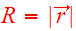and. The system hasdegrees of freedom, so the phase space hasdimensions.

The equations of motion can be derived by starting with the Lagrangian,

(1)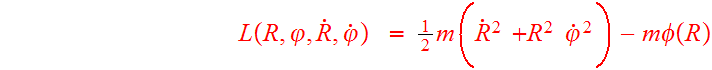,

whereand. In what follows, we will use a choice of unit mass andwill not show in the equations:

(2).

Differentiating with respect toandyields the momenta conjugate toand,

(3)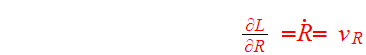,

(4)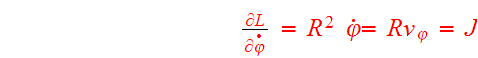,

whereandare velocities in the radial and azimuthal directions. The Hamiltonian (energy) may now be expressed as a function of the coordinates and conjugate momenta:

(5).

The equations of motions are

(6)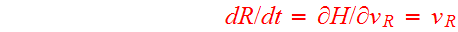,

(7),

(8),

(9).

Here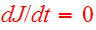because the conjugate coordinate phi does not appear in;is called a cyclic coordinate.

The system has two integrals of motion. One, of course, is the total energy, numerically equal to the value of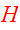. The other is the angular momentum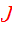. These quantities are given by

(10),

(11).

Each of these integrals of motion defines a hypersurface in phase space, and the orbit must remain in the intersection of these hypersurfaces. This can be visualized by ignoring thecoordinate and drawing surfaces of constantand J in the three-dimensional space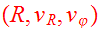. Surfaces of constantare figures of revolution about theaxis, while surfaces of constantare hyperbolas in theplane. The intersection of these surfaces is a closed curve, and an orbiting star travels around this curve.

For an orbit of a given, the system may be reduced to one degree of freedom by defining the effective potential,

(12)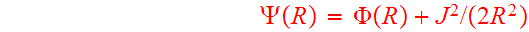;

the corresponding equations of motion are then just

(13),

(14)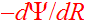.

Becausediverges as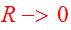, the star is energetically prohibited from coming too close to the origin, and shuttles back and forth between turning points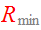and.

In addition to its periodic radial motion described by, a star also executes a periodic azimuthal motion as it orbits the center of the potential. If the radial and azimuthal periods are incommensurate, as is usually the case, the resulting orbit never returns to its starting point in phase space; in coordinate space such an orbit is a rosette. The Keplerian potential is a very special case in which the radial and azimuthal periods of all bound orbits are equal. The only other potential in which all orbits are closed is the harmonic potential generated by a uniform sphere; here the radial period is half the azimuthal one and all bound orbits are ellipses centered on the bottom of the potential well. Thus in the Keplerian case all stars advance in azimuth by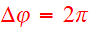between successive pericenters, while in the harmonic case they advance by. Galaxies typically have mass distributions intermediate between these extreme cases, so most orbits in spherical galaxies are rosettes advancing bybetween pericenters.

## N-body Equations of Motion

Any system in which stellar collisions are rare may be idealized as a collection of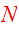point-sized bodies, each with mass, position, and velocity. The hamiltonian for such a system is

(15)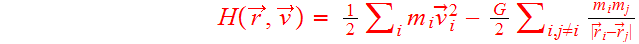,

wheredepends on all body positions and velocities, the first sum runs over allbodies, the second runs over all pairs of bodies (twice, hence the factor of), andis the gravitational constant. Then the equations of motion are

(16)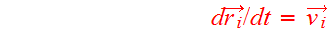,

(17)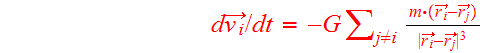,

where the sum runs over all bodies except body.

The famous Aarseth code in Fortran (Aarseth Fortran code) calculates the general motion of the N-body system in computer simulations.

N-body systems obey several basic conservation laws. A symmetry of the Hamiltonian is a transformation which leaves the physical system unchanged. For example, translation in time,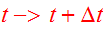, is a symmetry of Eq. 15 becauseis not an explicit function of time; consequently the total system energyis conserved. Likewise, symmetry with respect to translation in space, implies conservation of total linear momentum, and symmetry with respect to rotation gives rise to conservation of total angular momentum.

## Virial Parameters

Another general result shown by manipulating Eqs. 16,17 is the scalar virial theorem which states that for a system in equilibrium,

(18)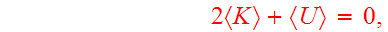where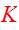and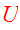are the total kinetic and potential energy, respectively, and the angle-brackets indicate time-averages. Since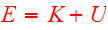, the time-averaged kinetic and potential energies are related to the conserved total energy by

(19).

The total massand total energy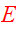of an N-body system thus define characteristic velocity and length scales

(20),

(21).

These are sometimes known as the virial velocity and radius, respectively.

The quantity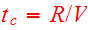is an estimate of the time a typical star takes to cross the system. This timescale may be expressed in several different ways; for example, in terms of the total massand energy, it is

(22).

Note thatandare conserved, sois a constant even for systems which are far from dynamical equilibrium. In such casesapproximates the time-scale over which the system evolves toward equilibrium.

Another expression forfollows from the substitutionvalid for systems near equilibrium:

(23).

Here the quantity, which has units of density, appears. In systems with galaxy-like density profiles, the virial radius is approximately proportional to the half-mass radius:. Using this relationship, it follows that

(23)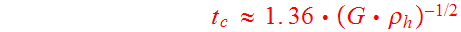,

where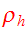is the mean density within. Since the crossing time is just supposed to indicate a typical time-scale for orbital motion, it is usual to drop the numerical constant, and define

(24).